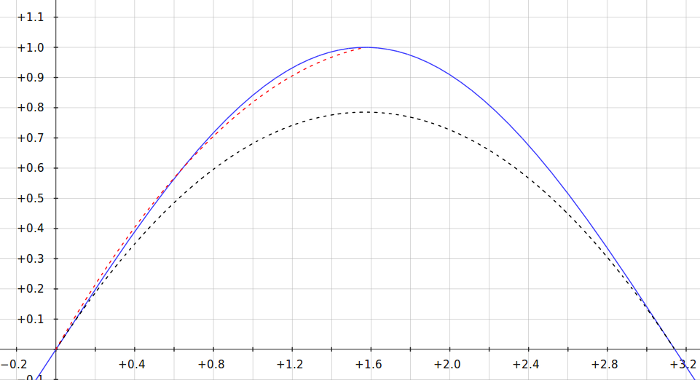While animating my first GADGET-2 galaxy mergers, I discovered that setting the frame rate to the common value of 25 frames per second resulted in movies that were too fast. The complexity of the merger process couldn’t be appreciated–even though the snapshot interval was quite small (0.005 simulation time units). I had to interpolate the GADGET-2 snapshots in order to produce intermediate frames that could be used to slow the animation down. Linear interpolation didn’t work well enough to meet my standards, so I looked for an efficient quadratic interpolation scheme. I wanted to perform a quadratic interpolation using only two snapshots at a time (a quadratic interpolation using two snapshots is possible in this case because each snapshot contains particle position and velocity information). The first thing that came to mind was simply using the familiar expression

$$!\displaystyle\mathbf{r}(t)=\mathbf{r}_0+\mathbf{v}t$$

to take a half-step forward from the first snapshot and a half step backward from the second snapshot and then average the two results together to estimate the likely position of each particle in the snapshot like this:

$$!\displaystyle\mathbf{r}_{1/2}=\frac{1}{2}(\mathbf{r}_0+\mathbf{r}_1)+\frac{1}{2}\left(\mathbf{v}_0\frac{\delta t}{2} – \mathbf{v}_1\frac{\delta t}{2}\right)$$

Where $$\delta t$$ is the time interval between snapshots $$0$$ and $$1$$, $$\mathbf{r}_{1/2}$$ is the position of a particle at time $$\delta t/2$$ after snapshot $$0$$, $$\mathbf{r}_0$$, $$\mathbf{r}_1$$, $$\mathbf{v}_0$$, and $$\mathbf{v}_1$$ are the positions and velocities of the particle at snapshot $$0$$ and snapshot $$1$$. This method is slightly better than linear interpolation, but not as good as using a quadratic spline. I tested it out and the results were disappointing. I searched my computational physics and numerical analysis textbooks and searched the web, but I couldn’t find what I was looking for, so I derived the method myself. My final result was $$!\displaystyle\mathbf{r}(t)=\frac{1}{\delta t}\left[\mathbf{r}_0(t_1-t)-\mathbf{r}_1(t_0-t)\right]+\frac{1}{2\delta t}\left(\mathbf{v}_1-\mathbf{v}_0\right)(t_1-t)(t_0-t)$$ This is very closely related to a quadratic Bézier curve. The derivation follows:

The Taylor expansion of a function $$f(x)$$ about the point $$x=x_0$$ is $$!\displaystyle f(x)=f(x_0)+(x-x_0)f'(x_0)+\frac{1}{2}(x-x_0)^2 f”(x_0)+\cdots$$

It is possible to approximate the value of the function at $$x_0$$ using a Taylor expansion by swapping $$x$$ with $$x_0$$ in the expansion above and truncating the series:

$$!\displaystyle f(x_0)\approx f(x)+(x_0-x)f'(x)+\frac{1}{2}(x_0-x)^2 f”(x)$$ Writing expansions for $$\mathbf{r}_0$$, $$\mathbf{r}_1$$, $$\mathbf{v}_0$$ and $$\mathbf{v_1}$$, $$!\mathbf{r}_{0}\approx\mathbf{r}(t)+(t_{0}-t)\mathbf{v}(t)+\frac{1}{2}(t_{0}-t)^2\mathbf{a}(t)$$ $$!\mathbf{r}_{1}\approx\mathbf{r}(t)+(t_{1}-t)\mathbf{v}(t)+\frac{1}{2}(t_{1}-t)^2\mathbf{a}(t)$$ $$!\mathbf{v}_{0}\approx\mathbf{v}(t)+(t_{0}-t)\mathbf{a}(t)$$ $$!\mathbf{v}_{1}\approx\mathbf{v}(t)+(t_{1}-t)\mathbf{a}(t)$$ Eliminating $$\mathbf{a}(t)$$ using the third and fourth equations: $$!\displaystyle\mathbf{a}(t)\approx\frac{\mathbf{v}_{1}-\mathbf{v}(t)}{(t_{1}-t)}\approx\frac{\mathbf{v}_{0}-\mathbf{v}(t)}{(t_{0}-t)}$$ The first two equations then become $$!\mathbf{r}_{0}\approx\mathbf{r}(t)+\frac{1}{2}(t_{0}-t)[\mathbf{v}_{0}+\mathbf{v}(t)]$$ $$!\mathbf{r}_{1}\approx\mathbf{r}(t)+\frac{1}{2}(t_{1}-t)[\mathbf{v}_{1}+\mathbf{v}(t)]$$ Now we eliminate $$\mathbf{v}(t)$$ between the two equations $$!\frac{2\mathbf{r}_{0}-2\mathbf{r}(t)}{(t_{0}-t)}-\mathbf{v}_{0}=\frac{2\mathbf{r}_{1}-2\mathbf{r}(t)}{(t_{1}-t)}-\mathbf{v}_{1}$$ Solving for $$\mathbf{r}(t)$$ and defining $$\delta t=t_1-t_0$$ yields the result stated above.

This is a decent approximation for the motion of each particle between snapshots. In fact, it’s a pretty good approximation for any function with specified end point values and first derivatives as long as the sign of the second derivative doesn’t change during the interval.

For demonstration purposes, the blue curve below is $$\sin(x)$$. The black dashed curve is the interpolating parabola generated by the expression derived above for endpoints at $$x_0=0$$ and $$x_1=\pi$$. The red dashed curve is the interpolating parabola for endpoints at $$x_0=0$$ and $$x_1=\pi/2$$Tags: ,

### One Response to “Quadratic Spline Interpolation”

1.bestessay Says:

A children who is interesting in the sports and he is studying some other courses so they never able to become successful and developed. The students should know that what courses they should learn and what the profession they should select in their life.